Games
Problems
Go Pro!

# Geometry

Lesson Plans > Mathematics > Geometry

# Featured Lesson Plans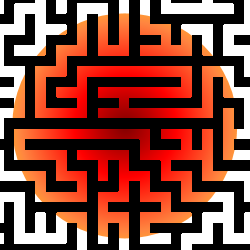The way definitions are written can either help or hinder our proof-writing skills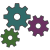How you can encourage some creativity in your geometry students, by suggesting they write their own theorems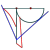Trisecting an angle is impossible with a straight-edge and compass, but a special tool called a 'tomahawk' makes this construction possible.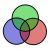How to help students understand how the words some, all, and none work together in logic statements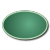A discovery based lesson in which students will use a graphing calculator to explore how the equation of an ellipse relates to its graph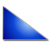A linear coordinate geometry math problem that can be solved in at least four different ways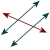Two very different way of looking at transversals - comparing them to goal posts and continentsSome thoughts about how we decide what we can assume, based on a diagram in a geometry problem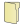Like us on Facebook to get updates about new resources